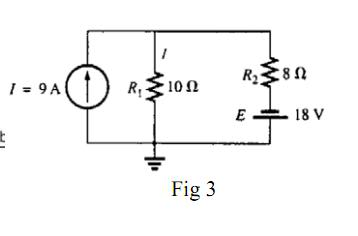# Current and voltage sources

## Homework Statement

Find the current through the resistor R1.## The Attempt at a Solution

First I short circuited the voltage source and determined I1.

I1=(9A)(10-olms/18-olms)=5 A down

Next, I short circuited the current source and determined the current going through R1 via olms law.

I2=18V/18-olm= 1 A up

Therefore, the total current flowing through the resistor R2 is 4 A down

Does this seem right?

## Answers and Replies

You made a mistake in finding I1 when you short circuited the voltage source. What i would do is to find the equivalent resistance of R1 and R2, then the voltage across them, and then I1.
Since R1 is bigger than R2 there should be more current through R2

The rest is OK.

There is more current going through R2 I thought.

I think I figured it out.

I1=(9A)(80)/(180) = 4 A down

Then that means that there is 3 amps down total. Does that seem more appropriate?

Anyone?

gneill
Mentor
3 amps looks good.